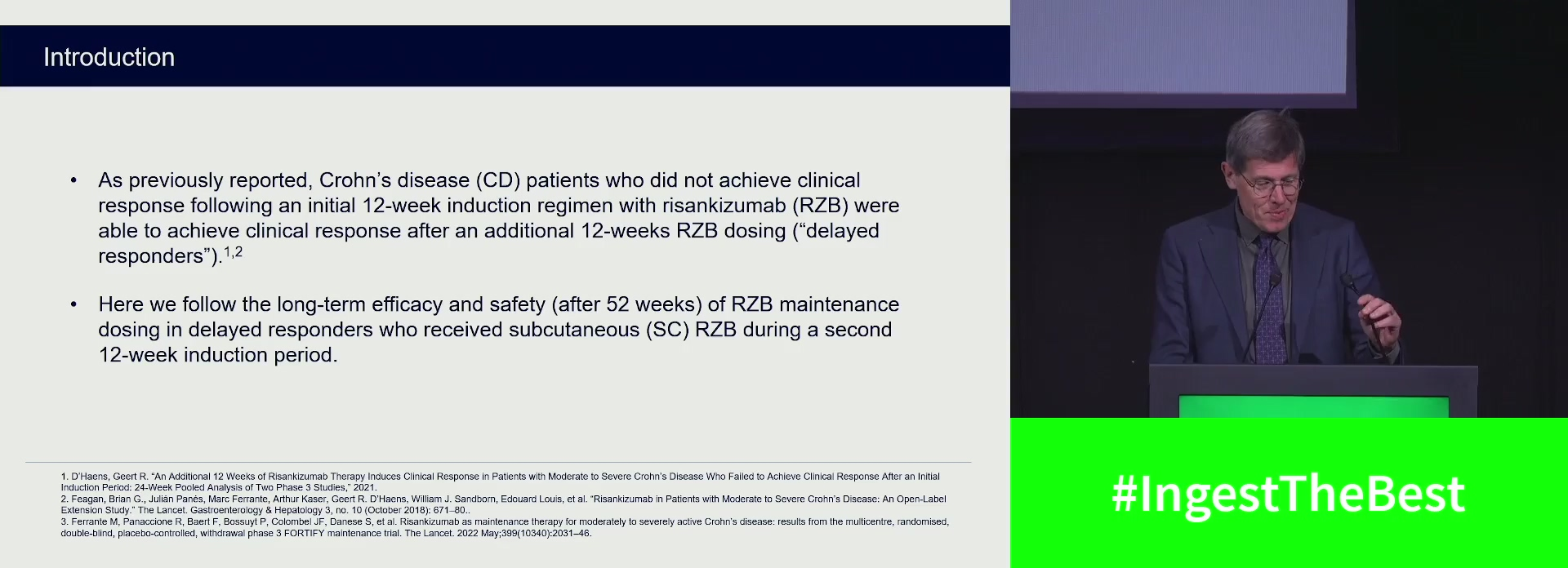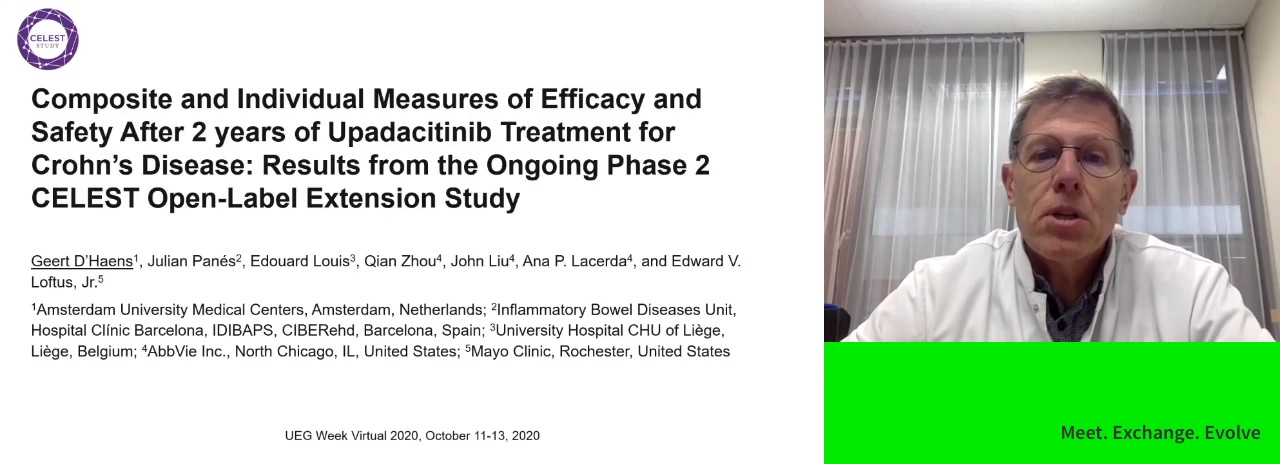### Geert R. D'HaensGeert R. D'Haens  et al.Geert R. D'Haens  et al.Geert R. D'Haens  et al.Geert R. D'Haens  et al.Geert R. D'Haens  et al.Geert R. D'Haens  et al.Geert R. D'Haens  et al.Geert R. D'Haens  et al.Geert R. D'Haens  et al.Geert R. D'Haens  et al.Geert R. D'Haens  et al.Geert R. D'Haens  et al.Geert R. D'Haens  et al.

Item 1 - 20 / 77